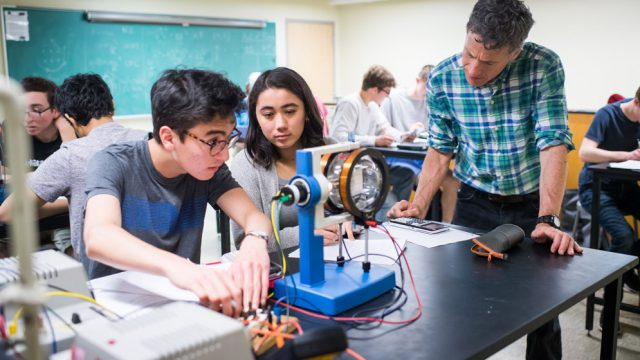## AP Physics: Strategies for Problem-SolvingThe AP Physics exams, both Physics 1 and Physics 2, assess your understanding of fundamental physics concepts and your problem-solving skills. To succeed in these exams, it's crucial to develop effective strategies for problem-solving. In this article, we'll explore some valuable strategies to help you tackle AP Physics problems with confidence.

## Understanding the AP Physics Exams

Before delving into problem-solving strategies, it's essential to understand the format and content of the AP Physics exams:

### - AP Physics 1:

This exam covers topics such as kinematics, dynamics, circular motion, work and energy, linear momentum, and simple harmonic motion.

### - AP Physics 2:

This exam includes concepts related to fluid dynamics, thermodynamics, electricity, magnetism, optics, and modern physics.

Both exams consist of multiple-choice questions and free-response questions. The free-response questions include both short and long questions that assess your ability to apply physics principles to solve problems.

## Strategies for Problem-Solving in AP Physics

### 1. Understand the Fundamental Concepts:

Before you can solve physics problems, you need a strong understanding of the underlying concepts. Review your textbook, class notes, and supplementary materials to ensure you grasp the principles.

### 3. Read the Problem Carefully:

Take your time to read the problem thoroughly. Understand what's given and what's being asked. Identify key variables and units.

### 4. Draw Diagrams:

For problems involving motion or geometric relationships, draw diagrams to visualize the situation. Label the relevant quantities on the diagram.

### 5. Apply Problem-Solving Strategies:

- For kinematics problems, use the equations of motion (e.g., the SUVAT equations).

- For dynamics problems, analyze forces, Newton's laws, and work-energy relationships.

- For electricity and magnetism problems, apply Ohm's law, Kirchhoff's rules, and electromagnetic principles.

- For optics problems, use ray diagrams and lens/mirror equations.

- For thermodynamics problems, apply the laws of thermodynamics and thermodynamic processes.

### 6. Unit Analysis:

Pay attention to units. Ensure that your equations and calculations are consistent with the required units. This is a powerful error-checking method.

### 7. Use the Right Formulae:

Use the appropriate equations and formulae for the given situation. Familiarity with the correct formulas is crucial.

### 8. Practice with Real Exam Questions:

Practice with official AP Physics questions from past exams. This will help you become accustomed to the format and style of questions you'll encounter on the actual test.

### 10. Check for Consistency:

Ensure that your answers and calculations are logically consistent. If an answer doesn't make sense based on the given conditions, it's likely incorrect.

### 11. Partial Credit:

On the free-response questions, remember that you can earn partial credit for showing your work. Even if you don't arrive at the final answer, demonstrating a clear and methodical approach can earn you points.